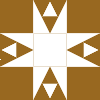2018-09-14 00:20:16

#### Analysis

1. 先找 $b$ 的个位，肯定只有 $1, 9$ 满足平方数末尾是 $1$
2. 再找 $b$ 的十位，用 0 ~ 9 十个数字拼上前面找的个位数，看看能不能得到 $21$
3. 再找 $b$ 的百位，用 0 ~ 9 十个数字拼上前面找的两位数，看看平方能不能得到 $321$
4. ...

#include <cstdio>
#include <vector>

using namespace std;

typedef long long INT64;

const INT64 tail = 987654321;

int main()
{
vector<int> nums;

nums.push_back(0);

vector<int>::iterator it;
int n;
for (int i = 1, base = 10; i < 10; ++i, base *= 10)
{
for (int d = 0; d < 10; ++d)
for (it = nums[i - 1].begin(); it != nums[i - 1].end(); ++it)
{
n = base / 10 * d + *it;
if ((INT64)n * n % base == tail % base)
{
printf("%d %lld\n", n, (INT64)n * n);
nums[i].push_back(n);
}
}
printf("\n");
}
}
• star
首页